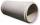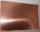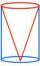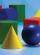# Solid geometry, stereometry

Solid geometry is the name for the geometry of three-dimensional Euclidean space.

Stereometry involves the measurements of volumes of various solid figures (three-dimensional figures), including pyramids, prisms, and other polyhedrons; cylinders; cones; truncated cones; and balls bounded by spheres.

#### Number of problems found: 1103

• Barrel of oilBarrel of oil weighs 283 kg. When it mold 26% oil, weighed 216 kg. What is the mass of the empty barrel?
• Concrete pipeConcrete pipe is cylindrical with an inner diameter 110 cm and outer 120 cm. Calculate the surface of the concrete pipe, if it is 9 m long.
• Copper plateCopper plate length 3.2 m and width 50 cm weighs 55.42 kg. What is the plate thick, if 1 m3 of copper weighs about 8700 kg?
• RollerCylinder shell has the same content as one of its bases. Cylinder height is 15 dm. What is the radius of the base of the cylinder?
• Cylinder - A&VThe cylinder has a volume 1287. The base has a radius 10. What is the area of the surface of the cylinder?
• Cylinder - basicsCylinder with base radius r = 54 cm and height h=35 cm. Calculate: a) Area of the base
• Sphere A2VThe surface of the sphere is 241 mm2. What is its volume?
• Sphere growthHow many times grow volume of sphere if diameter rises 10×?
• Sphere fallHow many percent fall volume of a sphere if diameter decreases 3×?
• Floating barrelBarrel (cylinder shape) floats on water, top of the barrel is 8 dm above water, and the width of surfaced barrel part is 23 dm. Barrel length is 24 dm. Calculate the volume of the barrel.
• PresentGift box has a rectangular shape with dimensions of 8×8×3 cm. Miloslav wants to cover with square paper with sides of 18 cm. How much paper left him?
• Cone in cylinderThe cylinder is inscribed cone. Determine the ratio of the volume of cone and cylinder. The ratio express as a decimal number and as percentage.
• Cone in cubeThe cube is inscribed cone. Determine the ratio of the volume of cone and cube. The ratio express as a decimal number and as percentage.
• AquariumAquarium is rectangular box with square base containing 76 liters of water. Length of base edge is 42 cm. To what height the water level goes?
• Cube wallThe surface of the first cube wall is 64 m2. The second cube area is 40% of the surface of the first cube. Determine the length of the edge of the second cube (x).
• Cube in ballCube is inscribed into the sphere of radius 181 dm. How many percent is the volume of cube of the volume of the sphere?
• Hollow sphereCalculate the weight of a hollow stříbrné sphere (density 10.5 g/cm3) if the vnitřní diameter is 15 cm and wall thickness is 1 mm.
• Cube - anglesCalculate angle between the wall diagonal and cube base. Calculate the angle between the cube body diagonal and cube base.
• Plasticine ballPlasticine balls have radius r1=85 cm, r2=60 mm, r3=59 cm, r4=86 cm, r5=20 cm, r6=76 mm, r7=81 mm, r8=25 mm, r9=19 mm, r10=14 cm. For these balls
• Cube diagonalDetermine the length of the cube diagonal with edge 37 mm.

Do you have an exciting math question or word problem that you can't solve? Ask a question or post a math problem, and we can try to solve it.

We will send a solution to your e-mail address. Solved examples are also published here. Please enter the e-mail correctly and check whether you don't have a full mailbox.

Please do not submit problems from current active competitions such as Mathematical Olympiad, correspondence seminars etc...